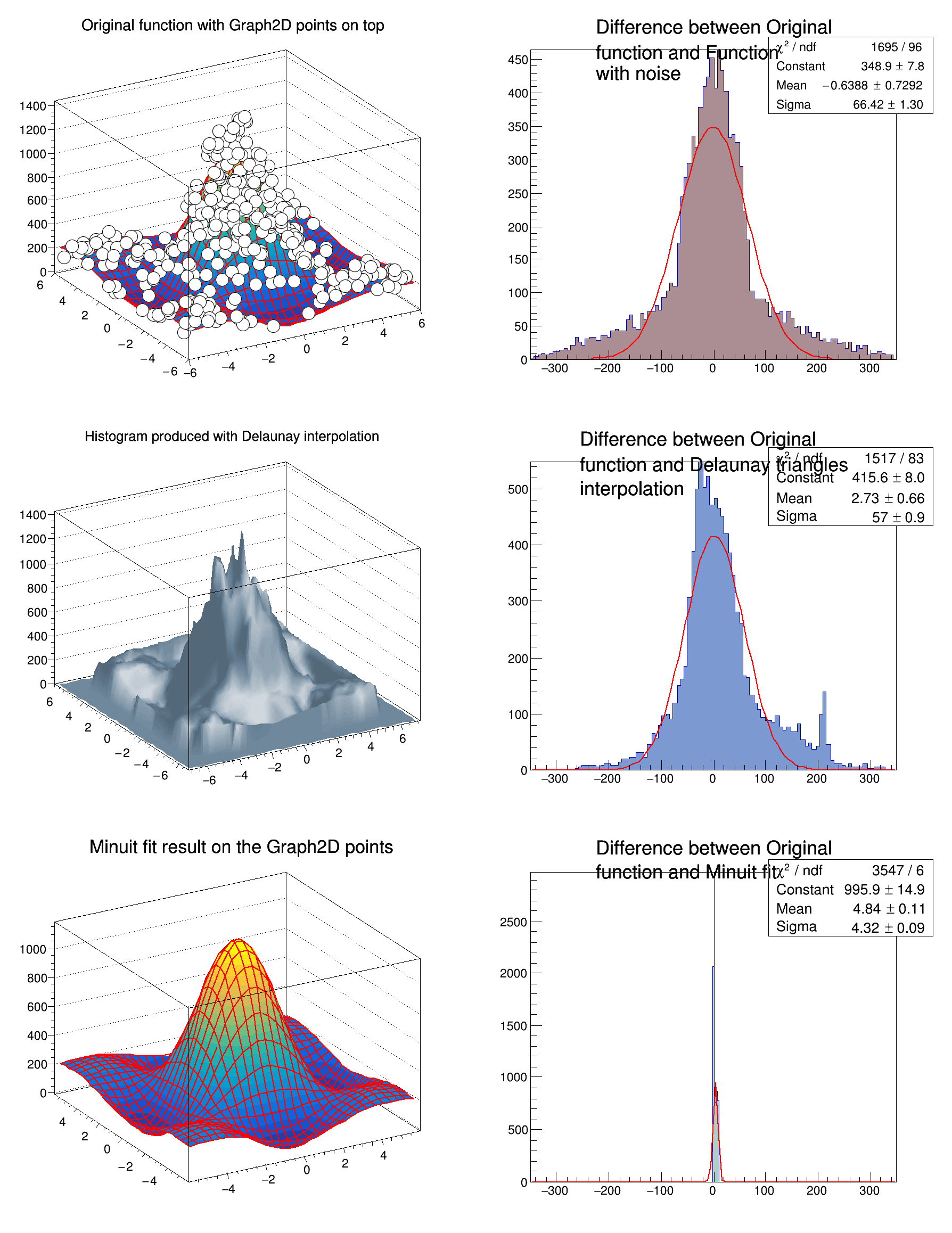ROOT   Reference Guide
Searching...
No Matches
graph2dfit.C File Reference

## Detailed Description****************************************
Chi2 = 4.25425e+06
NDf = 398
Edm = 1.32198e-12
NCalls = 55
p0 = 0.574556 +/- 0.109982
p1 = 1.72367 +/- 0.329947
(TCanvas *) 0x5589870b8810
#include <TMath.h>
#include <TGraph2D.h>
#include <TRandom.h>
#include <TStyle.h>
#include <TCanvas.h>
#include <TF2.h>
#include <TH1.h>
TCanvas* graph2dfit()
{
TCanvas *c = new TCanvas("c","Graph2D example",0,0,600,800);
c->Divide(2,3);
Double_t rnd, x, y, z;
Double_t e = 0.3;
Int_t nd = 400;
Int_t np = 10000;
Double_t fl = 6;
TF2 *f2 = new TF2("f2","1000*((*sin(x)/x)*(*sin(y)/y))+200",
-fl,fl,-fl,fl);
f2->SetParameters(1,1);
TGraph2D *dt = new TGraph2D();
// Fill the 2D graph
Double_t zmax = 0;
for (Int_t N=0; N<nd; N++) {
f2->GetRandom2(x,y);
// Generate a random number in [-e,e]
rnd = 2*r.Rndm()*e-e;
z = f2->Eval(x,y)*(1+rnd);
if (z>zmax) zmax = z;
dt->SetPoint(N,x,y,z);
}
Double_t hr = 350;
TH1D *h1 = new TH1D("h1",
"#splitline{Difference between Original}{#splitline{function and Function}{with noise}}",
100, -hr, hr);
TH1D *h2 = new TH1D("h2",
"#splitline{Difference between Original}{#splitline{function and Delaunay triangles}{interpolation}}",
100, -hr, hr);
TH1D *h3 = new TH1D("h3",
"#splitline{Difference between Original}{function and Minuit fit}",
500, -hr, hr);
f2->SetParameters(0.5,1.5);
dt->Fit(f2);
TF2 *fit2 = (TF2*)dt->FindObject("f2");
f2->SetParameters(1,1);
for (Int_t N=0; N<np; N++) {
f2->GetRandom2(x,y);
// Generate a random number in [-e,e]
rnd = 2*r.Rndm()*e-e;
z = f2->Eval(x,y)*(1+rnd);
h1->Fill(f2->Eval(x,y)-z);
z = dt->Interpolate(x,y);
h2->Fill(f2->Eval(x,y)-z);
z = fit2->Eval(x,y);
h3->Fill(f2->Eval(x,y)-z);
}
c->cd(1);
f2->SetTitle("Original function with Graph2D points on top");
f2->SetMaximum(zmax);
f2->Draw("surf1");
dt->Draw("same p0");
c->cd(3);
dt->SetMargin(0.1);
dt->SetFillColor(36);
dt->SetTitle("Histogram produced with Delaunay interpolation");
dt->Draw("surf4");
c->cd(5);
fit2->SetTitle("Minuit fit result on the Graph2D points");
fit2->Draw("surf1");
h2->SetFillColor(38);
h3->SetFillColor(29);
c->cd(2); h1->Fit("gaus","Q") ; h1->Draw();
c->cd(4); h2->Fit("gaus","Q") ; h2->Draw();
c->cd(6); h3->Fit("gaus","Q") ; h3->Draw();
c->cd();
return c;
}
ROOT::R::TRInterface & r
Definition Object.C:4
#define c(i)
Definition RSha256.hxx:101
#define e(i)
Definition RSha256.hxx:103
int Int_t
Definition RtypesCore.h:45
double Double_t
Definition RtypesCore.h:59
#define N
R__EXTERN TStyle * gStyle
Definition TStyle.h:412
virtual void SetFillColor(Color_t fcolor)
Set the fill area color.
Definition TAttFill.h:37
The Canvas class.
Definition TCanvas.h:23
virtual void SetMaximum(Double_t maximum=-1111)
Set the maximum value along Y for this function In case the function is already drawn,...
Definition TF1.cxx:3407
virtual void SetTitle(const char *title="")
Set function title if title has the form "fffffff;xxxx;yyyy", it is assumed that the function title i...
Definition TF1.cxx:3562
virtual void SetParameters(const Double_t *params)
Definition TF1.h:644
virtual Double_t Eval(Double_t x, Double_t y=0, Double_t z=0, Double_t t=0) const
Evaluate this function.
Definition TF1.cxx:1434
A 2-Dim function with parameters.
Definition TF2.h:29
virtual void GetRandom2(Double_t &xrandom, Double_t &yrandom, TRandom *rng=nullptr)
Return 2 random numbers following this function shape.
Definition TF2.cxx:529
virtual void Draw(Option_t *option="")
Draw this function with its current attributes.
Definition TF2.cxx:241
Graphics object made of three arrays X, Y and Z with the same number of points each.
Definition TGraph2D.h:41
Double_t Interpolate(Double_t x, Double_t y)
Finds the z value at the position (x,y) thanks to the Delaunay interpolation.
virtual TFitResultPtr Fit(const char *formula, Option_t *option="", Option_t *goption="")
Fits this graph with function with name fname Predefined functions such as gaus, expo and poln are au...
Definition TGraph2D.cxx:765
virtual TObject * FindObject(const char *name) const
search object named name in the list of functions
Definition TGraph2D.cxx:741
void SetMargin(Double_t m=0.1)
Sets the extra space (in %) around interpolated area for the 2D histogram.
virtual void SetTitle(const char *title="")
Sets the 2D graph title.
virtual void SetPoint(Int_t point, Double_t x, Double_t y, Double_t z)
Sets point number n.
virtual void Draw(Option_t *option="P0")
Specific drawing options can be used to paint a TGraph2D:
Definition TGraph2D.cxx:712
1-D histogram with a double per channel (see TH1 documentation)}
Definition TH1.h:618
virtual TFitResultPtr Fit(const char *formula, Option_t *option="", Option_t *goption="", Double_t xmin=0, Double_t xmax=0)
Fit histogram with function fname.
Definition TH1.cxx:3892
virtual Int_t Fill(Double_t x)
Increment bin with abscissa X by 1.
Definition TH1.cxx:3350
virtual void Draw(Option_t *option="")
Draw this histogram with options.
Definition TH1.cxx:3073
This is the base class for the ROOT Random number generators.
Definition TRandom.h:27
void SetOptStat(Int_t stat=1)
The type of information printed in the histogram statistics box can be selected via the parameter mod...
Definition TStyle.cxx:1589
void SetHistTopMargin(Double_t hmax=0.05)
Definition TStyle.h:368
void SetOptFit(Int_t fit=1)
The type of information about fit parameters printed in the histogram statistics box can be selected ...
Definition TStyle.cxx:1541
Double_t y[n]
Definition legend1.C:17
Double_t x[n]
Definition legend1.C:17
TH1F * h1
Definition legend1.C:5

Definition in file graph2dfit.C.